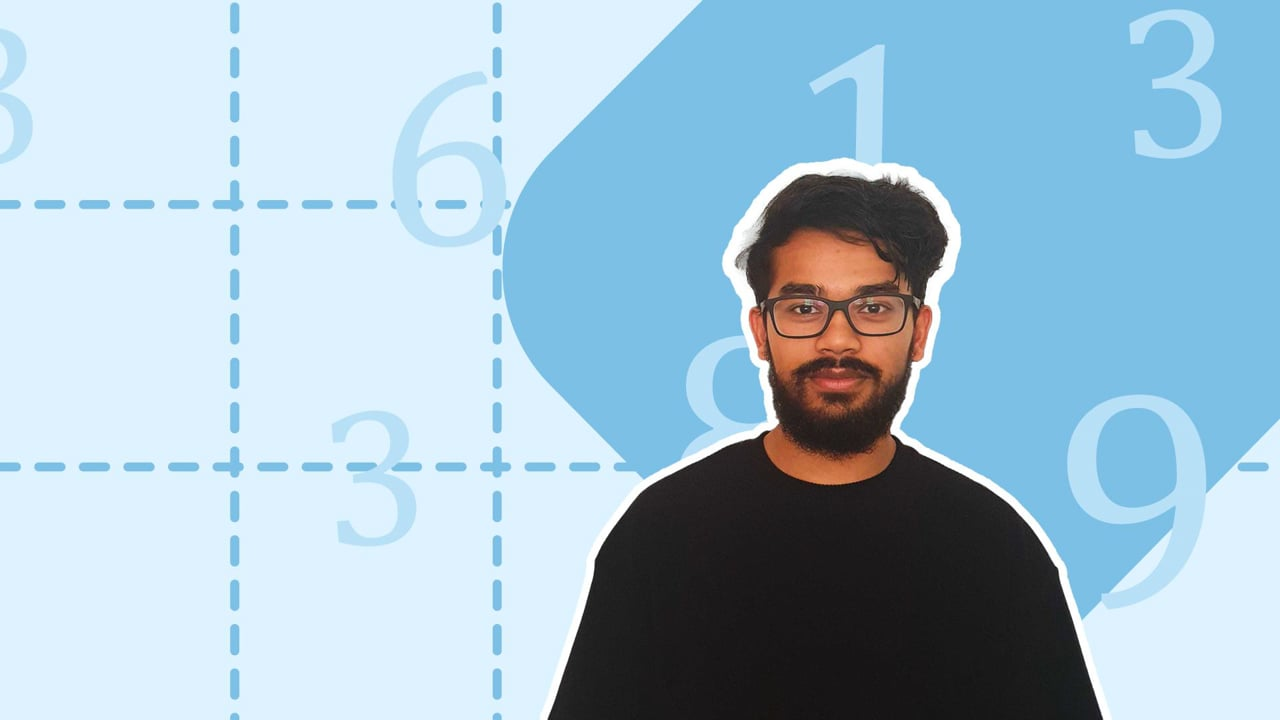Chapter overviewMaths

Number and place value

Multiplication and division

Fractions

Measurement

Geometry - properties of shapes

Geometry - position and direction

Statistics

Ratio and proportion

Algebra

Maths

# Using estimation to check answers0%

Summary

# Using estimation to check answers

## ​​In a nutshell

Estimation involves finding an approximate answer for a calculation so you can have an idea that the real answer is correct. The main method of estimation is to round numbers in a calculation to make it faster and easier to perform the calculation.

## Estimating

When estimating a calculation, round the numbers so they are easier to handle. Round the numbers to the first digit, then the calculation should be easier to perform. To represent an estimation, use the symbol $(\approx)$ which means "approximately equal to".

##### Example 1

Estimate $612 \times 11$.

First, round $612$.

$612 \approx 600$

Then, round $11$.

$11 \approx 10$

Attempt the calculation with the rounded numbers.

$600 \times 10 = 6000$

Therefore,

$\underline{621 \times 11 \approx 6000.}$

Note: You may not need to round every number in a calculation as long as you are still able to estimate a calculations.

##### Example 2

Estimate $7141- 3987$.

Round $7141$ and $3987$ using the first digit.

$7141 \approx 7000 \\3987 \approx 4000$

$7000-4000 =3000$

Therefore,

$\underline{7141-3987 \approx 3000.}$​​

FAQs

• Question: How should you round numbers when estimating a calculation?

Answer: Numbers can be rounded when estimating a calculation. Round the numbers in the calculation to the first digit. E.g. To find 713 x 62, round 713 to 700 and round 62 to 60.

• Question: What is the purpose of estimation?

Answer: Estimation involves finding an approximate answer for a calculation so you can have an idea that the real answer is correct.

• Question: How do you estimate numbers?

Answer: The main method of estimation is to round numbers in a calculation to make it easy to answer.

Theory

Exercises